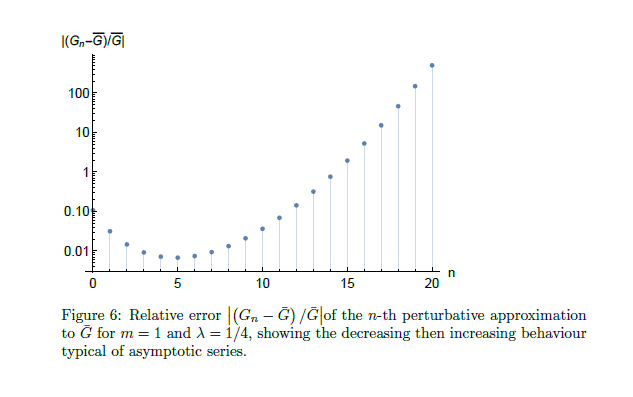## Tuesday, September 1, 2020

### High Energy Physics Quote Of The Day

Quantum chromodynamics (QCD) calculations have been lagging behind experimental tests of it in precision for the last 40 years, at least. Feynman concluded his short introduction to quantum mechanics, "QED" (1985) with almost the same observation.
When particle physicists try to model experiments, they confront an impossible calculation — an infinitely long equation that lies beyond the reach of modern mathematics.
Fortunately, they can generate largely accurate predictions without seeing this arcane math all the way through. By cutting the calculation short, scientists at CERN’s Large Hadron Collider in Europe make forecasts that match events they actually observe when they send subatomic particles barreling toward each other around a nearly 17-mile track.
Unfortunately, the era of agreement between forecast and observation may be ending. As measurements grow more precise, the approximation schemes theorists use to make predictions may not be able to keep up.
From here

I explained the issue at greater length in a recent comment at the Physics Forums:
QCD calculations are not very precise, and this isn't just a matter of imprecise measurements.

For example, we have measured the mass of the charged pion to a precision of 1.5 parts per million. But we can only calculate that mass from first principles to a precision of a few parts per thousand.

We understand why this is the case quite well. The strong force coupling constant has been measured only to about one part per thousand. The high numerical value of the dimensionless strong force coupling constant relative to one means that even at five loops you only have about one part per thousand theoretical precision compared to one part per 61 billion theoretical precision in QED calculations to five loops in which the dimensionless QED coupling constant is much smaller relative to one. Photons don't have self-interactions and only have one charge plus polarization, greatly reducing the number of possible Feynman diagrams per loop of calculation and qualitatively reducing the potential complexity involved, while gluons have self-interactions and three colors in addition to polarization requiring far more Feynman diagrams per loop. We can observed free photons and charged particles, while quarks and gluons (apart from top quarks) are confined so we have to infer their properties from composite particles.

But the problem of the slow convergence of the infinite series path integrals we use do to QCD calculations isn't simply a case of not being able to throw enough computing power at it. The deeper problem is that the infinite series whose sum we truncate to quantitatively evaluate path integrals isn't convergent. After about five loops, using current methods, your relative error starts increasing rather than decreasing. From the link in this paragraph:When you can get to parts per 61 billion accuracy at five loops as you can in QED, or even to the roughly one part per 10 million accuracy you can in weak force calculations to five loops, this is a tolerable inconvenience since our theoretical calculations still exceed our capacity to measure the phenomena that precisely. But when you can only get to parts per thousand accuracy at five loops as is the case in perturbative QCD calculations, an inability to get great precision by considering more loops is a huge problem when it comes to making progress.

You can't even measure the fundamental constants with more accuracy due to this problem, because you do that by theoretically reverse engineering the precisely measured observable quantities of composite particles like the charged pion and proton and neutron masses, with QCD theoretical formulations, to figure out the values of the fundamental constants that produce those experimental values in the theory.

Now, this isn't necessarily insurmountable. It might be possible to use math tricks to do better. For example, mathematically speaking, one can resort to techniques along the lines of a Borel transformation to convert a divergent series into a convergent one. And, path integral formulations are limitations associated with perturbative QCD calculations that can be overcome by using non-perturbative QCD methods like lattice QCD. But getting great precision in QCD calculations is as much a theoretical problem as a practical one.